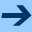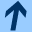Introduction

# Introduction

RAPTURE is a verification tool developped jointly by BRICS and INRIA. The tool is designed to verify reachability properties on Markov Decision Processes (MDP), also known as Probabilistic Transition Systems. This model can be viewed both as an extension to classical (finite-state) transition systems extended with probability distributions on successor states, or as an extension of Markov Chains with non-determinism.

We have developped a simple automata language that allows to describe a set of processes communicating over a set of channels ŕ la CSP. Processes can also manipulate local and global variables of finite type.

The properties are specified by defining two sets of initial and final states in the description of the automaton, and by defining the type of the property on the command line of the tool.

The originality of the tool is to provide two reduction techniques that limits the state space explosion problem: automatic abstraction and refinement algorithms [DJJL01], and the so-called essential states reduction [DJJL02]. Another original feature is to provide the choice between two LP solvers for the numerical computations: the first one uses sparse matrix on floating point numbers, the second uses dense matrix on exact rational numbers, which enable exact computations.

The tool has been developped mainly in Caml, and uses the BDD library Cudd and the LP solvers LPsolve and CddLib.

We have used RAPTURE on a number of case studies, one of which can be found here in a simplified version

The tool can be downloaded in binary format for Linux and Solaris systems. A tutorial is available, as well as a user manual.

Bertrand Jeannet, July 18, 2006Introduction